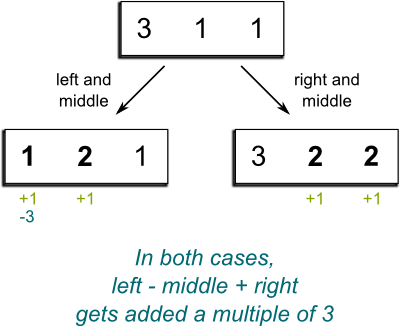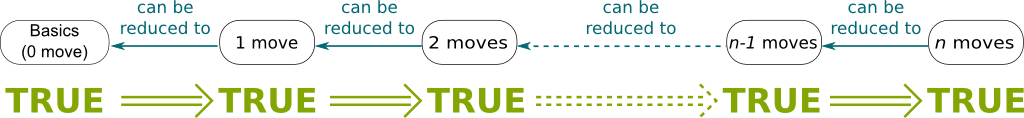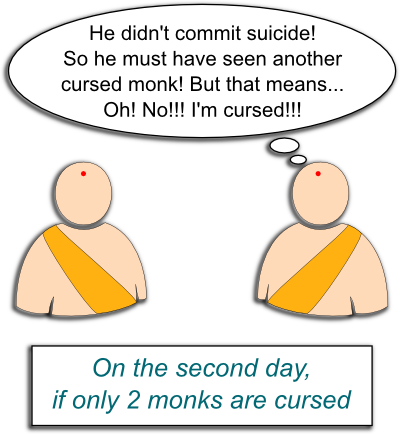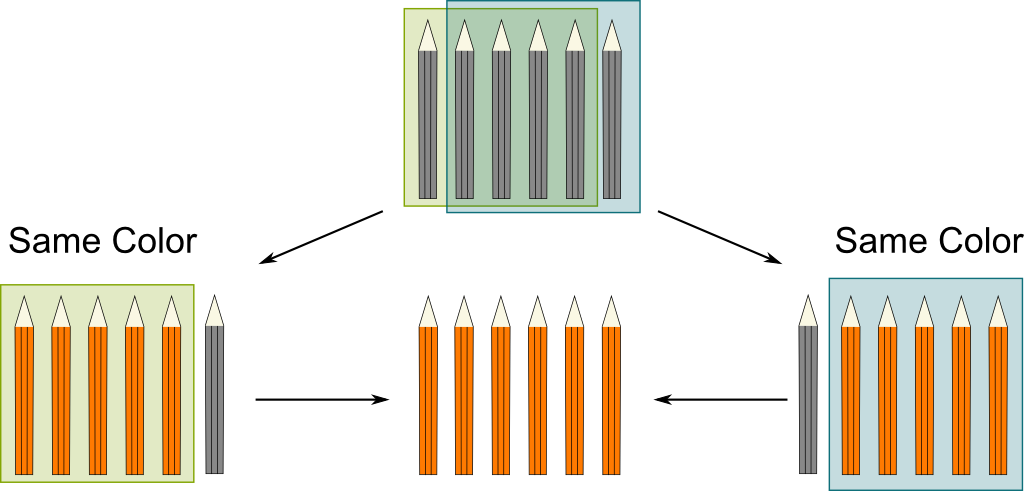# Proof by Mathematical InductionIn the savannah, lions are starving and merciless. Each one values his own life more than anything else, and is willing to eat another lion if it can. Suddenly a sick zebra falls dead in the middle of the savannah. Will lions come and eat it?

They’re starving, aren’t they?

Yes, but once a lion starts feeding, it becomes vulnerable to other lions’ attacks… Also, I should mention that in this particular savannah, lions have high IQs!

Then I guess that none of them eats the dead zebra…

Take your time to meditate on this problem…

Humm… Can you give a clue?

Let me give you the basics of proof by induction. This should help you with the lion problem.

## A Lock Puzzle

So what’s a proof by induction?

Let me start with a classical math joke. Assuming it takes 3 physicists to screw a lightbulb, one holding the lightbulb while the two others rotate the Universe (if you didn’t get it, check my article on space deformation!), how many mathematicians does it take to do the same job?

Humm…

Just the one. The mathematician hires 3 physicists and thereby reduces the problem to an earlier solved one.

Haha… Mathematicians love reducing problems to simpler ones!

Yes! Just like computer scientists like to use recursion! The idea is to first build up the basics. Then, the key is to find a way to reduce any problem to the basics.

Can you give an example?

Sure! Let’s consider this following problem by the amazing James Grime on SingingBanana.

Basically, with a specific 3-digit lock with numbers between 1 and 3, you can only turn the two first numbers simultaneously, or the two last simultaneously. Starting at combination 3-3-3, how can you get to 2-2-1?

James Grime posted a solution, but I’m going to give another solution to this puzzle, which I personally find even more elegant than his! More interestingly, mine involves proof by induction.

How does it work?

Hehe… We’re going to need to be a bit shrewd here. Let me claim that, no matter what moves we do, if we add the first and third numbers, and we subtract the second one, we always obtain a multiple of 3. In other words, $left-middle+right$ is divisible by 3. Let’s call this claim the invariance property. We can prove this by induction!

How do we do that?

Come on! I’ve just told you!

Oh yeah! First, we build up the basics… So that’d be the combination 3-3-3, right?

Yes! For this combination, we have $left-middle+right=3-3+3=3$. This is a multiple of 3!

That was easy… Now we have to reduce every combination to its basics?

Yes!

How do we do that?

Well, any combination you can reach is reached with a certain number of moves, right? Now, the combination 3-3-3 corresponds to 0 move, and we want to make sure that no matter what number of moves we do, we can always reduce our problem to the basic case. There are several ways to do so, but to highlight the most common approach to proofs by induction, let’s analyze the recursion property.

What’s the recursion property?

The recursion property consists in reducing the case of $n$ moves to cases of strictly smaller numbers of moves. In other words, we need to prove that, provided that the $(n-1)$-move case satisfies the invariance property, the $n$-move case does too.So how does this apply in our case?

First, we assume that any combination obtained after $n-1$ moves or less is such that $left-middle+right$ is a multiple of 3. This is known as the recursion hypothesis.

OK. What now?

Now, if we take a combination obtained after $n$ moves, then it is reached from a combination obtained after $n-1$ moves, by making another move. The recursion hypothesis tells us that the combination obtained after $n-1$ satisfies the invariance property, that is, $left-middle+right$ is a multiple of 3. Now, if we make the last move, then we’re either adding 1 to the left and middle numbers (and maybe retracting 3 if numbers go to 4) or adding 1 to the middle and right numbers (with the same remark). In either case, overall, we have added a multiple of 3 to the quantity $left-middle+right$. Thus, it is still a multiple of 3.

And this proves the recursion property, right?

Yes! The recursion property says that the invariance property for $n$ moves can thus be reduced to the invariance property for $n-1$ moves. But then, these combinations can be reduced to $n-2$, then for $n-3$, and so on… Until…

the basic case with no move!

Precisely! The following figure illustrates this inductive reasoning:This scheme shows how that the invariance property holds for all numbers of moves. Thus, for all reachable combinations!

The fact that this property indeed holds is intrinsically related to the existence of natural numbers, which is stated by Peano axioms. Find out more with my article on the construction of numbers!

An appropriate illustration of this recursion mechanism is the falling of dominos. By making sure that every domino falls, providing that the previous one does, and by making the first domino fall, all will fall eventually:

With this metaphor, proof by induction consists in two steps. First, we need to make sure that the first domino will fall. This corresponds to the basic case. Then, we need to check whether all dominoes are perfectly alined, such that every domino will make the next one fall. This stands for the recursion property.

So all reachable combinations satisfy the invariance property… What does it tell us regarding the lock puzzle?

If we apply this reasoning to James Grime’s puzzle, then you can see that the combination we are trying to achieve, 2-2-1, does not satisfy the invariance property. Indeed $2-2+1=1$, which is not divisible by 3! Thus, it cannot be reached! Hehe, James Grime, you cunning English mathematician…

Here are other interesting, although much easier, puzzles: What are all the possible reachable combinations? In particular, can all combinations satisfying the invariance property be reached? And for mathematicians: What is the orbit of reachable combinations isomorphic to? This last question has to do with group theory. You can learn more on this by reading my articles on symmetries and on space deformation and group representation.

And, now, we’re back to the lions! I’m going to give the answer. Try to find it yourself before reading it!

## Back to the LionsSo, have you figured it out?

Humm… I’m still thinking…

That’s it! I give up!

As I said, you need to apply the reasoning by induction I’ve presented. So, first…

First, I need to solve the basic case. Which corresponds to 1 lion, I guess…

Yes! What happens with 1 lion?

Obviously, he goes and eats the zebra, as he doesn’t need to fear any other lion.

Yes. What happens with 2 lions?

None should go, as both fear each other.

You’re on a roll! Keep it up! What happens with 3 lions?

Same thing as for 2 lions!

Are you sure? Let me rephrase my question. Can the 3-lion case be reduced to the 2-lion case?

Humm… Yes! I got it! If a lion goes and eats the zebra, then he sort of becomes the zebra!Bravo! And this reduces the problem to the 2-lion case! Let me recall you that in this 2-lion case, no lion would go and eat the zebra, so the lion who eats the zebra in a 3-lion case is safe! Now, what about the 4-lion case?

If a lion eats the zebra, then we’re back at the 3-lion case and the eating lion gets eaten. So no lion eats the zebra, right?

There you go! Let me recapitulate, in a 1-lion case, the lion eats the zebra. In a 2-lion case, no lion does. In a 3-lion case, a lion does. In a 4-lion case, no lion does. Do you see the pattern?

So, if there’s an even number of lions, then no lion eats the zebra. Otherwise, one does. Is that it?

Well, we now need to prove it. And we’ll do this by induction.

The basic cases work perfectly. So I guess we only need to show the recursion property…

Yes! So let’s assume your claim to be true for $n-1$ lions, and let’s consider $n$ lions. We need to distinct the cases where $n$ is even or odd.

Sure! Let’s start with the case where $n$ is even!

OK. If $n$ is even and a lion goes and eats the zebra, then he sort of becomes the zebra with $n-1$ lions around. But $n-1$ is then odd, and, according to the recursion hypothesis, he’d get eaten. So, when $n$ is even, no lion goes and eats the zebra!

Now, if $n$ is odd…

Then a lion that goes and eats the zebra would be surrounded by $n-1$ lions. Since $n-1$ is now even, he’d be safe. So, when $n$ is odd, one lion does go and eat the zebra! That’s it! We have proven the case of $n$ lion by reducing it to a problem of $n-1$ lions! The recurrence property is satisfied!

Yoohoo! So it does work! A lion eats the zebra if and only if there is an odd number of lions!

Problem solved! Let’s move on to a trickier one.

## Now to the Monks

In a monastery, 77 extraordinarily smart monks meet once a day to pray at noon. Although they see each other, they don’t talk nor communicate by any possible means. The monastery also has a strict no-mirror policy. A morning, a curse suddenly strikes the monastery. At least one monk is cursed. Monks know it. Cursed monks have a red dot on their forehead. But each has no idea whether he is cursed. As soon as one finds out he is cursed, for the good of the community, he must commit suicide at 7pm. Nothing occurs during the first 7 days. But on the evening of the 7th day, some monks commit suicide. Why?

To avoid spoiling you with the answer right here, here’s a picture I took in Ayutthaya in Thailand. The answer to the monk problem is then given below.So, have you figured it out?

I’ve tried to take the basic case of 1 smart monk in the monastery but it doesn’t work!

You’re not doing the induction with the right variable!

I’ve also tried with the number of days before the monks commit suicide, but it still doesn’t work!

Think of the simplest case! This is the case where only one monk is struck by the curse.

Because he doesn’t see any cursed monk and knows that at least one is cursed, he commits suicide on the first evening, right?

Exactly! Let’s move on to the 2-cursed-monk case. Let’s call them Albert and Bob. On the first day, each sees another cursed monk. So none commits suicide. But on the next day, both discover that the other cursed monk is still alive and didn’t commit suicide. So…

each knows that the other has seen at least another cursed monk!Yes! Albert thus figures out that there is a cursed monk other than Bob. But he sees none. So it must be himself! Similarly, Bob figures out that he is cursed too. Therefore…

On the evening of the 2nd day, both thus commit suicide!

Exactly! So, when there’s one cursed monk, the suicide occurs on the first day. When there are 2, it occurs on the 2nd day.

So I guess that if $n$ monks are cursed, the suicide would occur on the $n$-th day. Right?

Prove it!

Sure! The basic cases have been dealt with. All that’s left is to prove the recursion property!

Yes. So, assume that your claim is true of $n-1$ monks, and prove it for $n$.

Easy! Each cursed monk sees $n-1$ cursed monks and thus expect the suicide to occur on the $(n-1)$-th day. But, no suicide occurrs. Thus, the cursed monks figure out that there are strictly more than $n-1$ of them. And they know that they are cursed!

Bravo! So, they all commit suicide on the $n$-th day. This proves the recursion property!

Woohoo! And thus, there were 7 cursed monks!

## The Pencil Conundrum

Now, to wrap up this article, let me finish with a disturbing reasoning. I’m going to prove by induction that no matter how many pencils you have, they are always of the same color!

That’s nonsense!

Wait for the proof! First, the basic case is obviously when you only have one pencil. It’s obviously of the same color of all the pencils I have.

So far so good…

Now, assume I have $n$ pencils. Let me leave one out. Then I have $n-1$ pencils. So I can apply the recursion hypothesis to these $n-1$ pencils, which are thus of the same color. But wait, let me take another pencil out, and pick again the pencil I initially left out. I still have $n-1$ pencils, and, according to the recursion hypothesis, they still are of the same color. Thus, all of them are of the same color! The recursion property is proven!

I’m troubled…

This is illustrated in the figure below. Now, obviously, there is something wrong in my proof. I challenge you to find it out!

Below the figure is the solution.Any luck?

Arg… I don’t see what’s wrong!

In fact, everything works perfectly for large numbers. But, sometimes in mathematics, like for Poincaré conjecture, the small numbers are the problems… Do you see what I mean?

Yes! I get it! The recursion property does not hold for 2 pencils!

Exactly! Each subgroup of pencils is of the same color. But, this color is not necessarily the same because there is no pencil belonging to both subgroups! In other words, all the dominoes are well aligned and ready to fall for $n \geq 3$, and even though the first domino does fall, the first domino doesn’t make the second fall. And all the other dominoes won’t fall.## Let’s Sum Up

Proof by induction was one of these essential ideas of logics and mathematics. It’s a very powerful technic, especially in constructive mathematics. I hope I have made you see why. This technic really relies on two aspects: The basic case and the recursion property. This idea is also the basis of recursion algorithms and structures, which I talk about in my article on self-reference.

Also, if you have other great puzzles involving proofs by induction, I’d love to add them here. Please send them to me!

## More on Science4All

Self-Reference, Math Foundations and Gödel's IncompletenessBy Lê Nguyên Hoang | Updated:2016-02 | Views: 6513
Although highly appreciated by artists, self-reference has been a nightmare for mathematicians. It took one of the greatest, Kurt Gödel, to provide a better understanding of it. This article deals with paradoxes, recursion, fractals and Gödel's incompleteness theorems.

Euler's Formula and the Utilities ProblemBy Lê Nguyên Hoang | Updated:2016-01 | Views: 18085
I was a kid when I was first introduced to the deceptively simple utilities problem. It's only lately that I've discovered its solution! And it's an amazing one! Indeed, it provides a wonderful insight into some fundamental mathematics, including Euler's formula! This is nothing less than the gateway to the wonderful world of algebraic topology!

Construction and Definition of NumbersBy Lê Nguyên Hoang | Updated:2016-02 | Views: 9073
Although they have been used for thousands of years, an actual definition of numbers was given less than a century ago! From the most fundamental level of set theory, this article takes you to the journey of the construction of natural, integer, rational, real and complex numbers.

Type Theory: A Modern Computable Paradigm for MathBy Lê Nguyên Hoang | Updated:2016-01 | Views: 12739
In 2013, three dozens of today's brightest minds have just laid out new foundation of mathematics after a year of collective effort. This new paradigm better fits both informal and computationally-checkable mathematics. There is little doubt that it will fundamentally change our perspective on rigorous knowledge, and it could be that, in a few decades, the book they published turns out to be the bedrock of all mathematics, and, by extension, all human knowledge! Have a primer of this upcoming revolution, with this article on type theory, the theory that the book builds upon!

## More Elsewhere

Mathematical Induction on Math is Fun.

1.Herminio López says:

The day I get lost in the savanna, I hope to meet an even number of lions.

And above all, I hope the lions know the proof by induction… 😉

Nice article!

2.memesworth says:

The lion example doesn’t work. All lions are equal so in the 3 lion example every lion should (according to your logic) approach the zebra to feed. You can’t have a unique lion. You postulate the existence of a state which can’t be reached.﻿

1.Lê Nguyên Hoang says:

Let’s say that they are randomly distributed geographically.

3.filipbacic says:

About that last example with pencils, how to recognize that kind of problem when it is not so obvious like for pencils? What are the additional constraints or requirements?

1.Lê Nguyên Hoang says:

First, allow me to say that I had a lot of trouble finding out what was wrong in this problem. Finding mistakes in mathematical proofs (like finding bugs in a computer code) can be a huge pain in the ass…
The key to recognizing any kind of problem in mathematics is rigour. I myself am not rigorous in these Science4All articles, and that’s a great way to disguise such problems as the pencil problem. Serious mathematics has to be rigorous, and that means that it involves cumbersome notations. These are hard to read, but if there’s a mistake somewhere, they’ll underline it clearly. That’s why rigorous formal mathematics is so important.

4.jakedid says:

Be sure the monk example starts out with seven monks! Not seventy-seven!